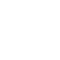# Forced Vibrations with Harmonic ExcitationSo far, we have discussed the free vibrations of systems where a system once disturbed from its equilibrium position executes vibration indefinitely because of its elastic properties. In actual practice, this system will come to rest, sooner or later, depending upon the amount of damping present in the system. In case of forced vibrations, the amplitude of vibration is almost constant due to the application of external forces. That is, when the body vibrates under the influence of external force, then the body is said to be under forced vibrations.

### Examples of Forced Vibrations

1.  Ringing of electrical bell where the vibration is by means of electrical means (electric supply is an external force).
2. The vibrations of air compressors, internal combustion engines, machine tools and various other machinery.

#### Types of External Excitation

Three types of external forces applied are

• Periodic forces,
• Impulsive type of forces,
• Random forces.

Periodic forces can be further categorised into harmonic and non-harmonic forces. Vibrations because of impulsive forces are called transient. Earthquake and acoustic excitations are typical examples of random forces. But the scope of this text is limited only to the analysis of periodic forcing functions.

#### Forced Vibrations with Constant Harmonic Excitation – [Harmonic Forcing]

Consider a spring mass system with viscous damping [Fig (a)], excited by a sinusoidal harmonic force F = Fo sin ωt.

At any instant, when the mass is displaced from the mean position through a distance x in the downward direction, the external forces acting on the system are shown in Fig (c).

These forces are:

1. Inertia force $=m \frac{d^{2} x}{d t^{2}}$ (upwards)
2. Damping force $=c \frac{d x}{d t}$  (upwards)
3. Spring force = s . x  (upwards)
4. Impressed (or external disturbing) force, F = Fo sin ωt (downwards)

According to D’Alemberts principle,

Inertia force + Σ External forces = 0

$\begin{array}{l} \\ m \frac{d^{2} x}{d t^{2}}+\left[c \frac{d x}{d t}+s x-\mathrm{F}_{\mathrm{O}} \sin \omega t\right]=0 \\ \qquad m \frac{d^{2} x}{d t^{2}}+c \frac{d x}{d t}+s x=\mathrm{F}_{\mathrm{O}} \sin \omega t \end{array}$

$\frac{d^{2} x}{d t^{2}}+\left(\frac{c}{m}\right) \frac{d x}{d t}+\left(\frac{s}{m}\right) x=\left(\frac{F_{0}}{m}\right) \sin \omega t$

This is the governing or characteristic equation of damped forced vibrations. This is a linear, non-homogeneous, second order differential equation. The solution of this equation consists of two parts: (i) complementary function (xc) and (ii) particular integral (xp).

∴ Complete solution x = xc + xp

#### To obtain Complementary Function (xc)

The complementary function is obtained from the differential equation (taking RHS value zero).

$m \frac{d^{2} x}{d t^{2}}+c \frac{d x}{d t}+s x=0$

This complementary function is same as the equation obtained for damped free longitudinal system. Therefore the solution of this equation has been already obtained and is given by

$\begin{array}{l} {x_{c}}=\mathrm{X} e^{-\zeta \omega_{n} t} \sin \left(\omega_{d} t+\phi\right) \\ x_{c}=\mathrm{X} e^{-\zeta \omega_{n} t} \sin \left[\sqrt{1-\zeta^{2}} \omega_{d} t+\phi\right] \end{array}$### About the author#### Santhakumar Raja

Hi, This blog is dedicated to students to stay update in the education industry. Motivates students to become better readers and writers.

View all posts